# How to do “Limitless” Math in Python

How to perform arbitrary-precision computation and much more math (and fast too) than what is possible with the built-in math library in Python.Image sourcePixabay (Free to use)

## Limitless math?

Sounds like a catchy title? Well, what we really meant by that term is arbitrary-precision computation i.e. breaking away from the restriction of 32-bit or 64-bit arithmetic that we are normally familiar with.

Here is a quick example.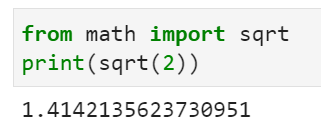This is what you will get as the value for the square-root of 2 if you just import from the standard `math` module of Python.

You can use Numpy to choose if you want the result to be 32-bit or 64-bit floating-point number.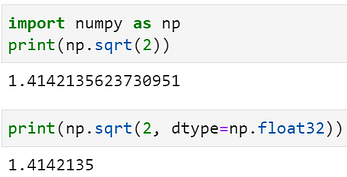But what if you wanted the result up to 25 decimal places…

1.414213562373095048801689

Or, 50 decimal places?

1.4142135623730950488016887242096980785696718753769

How are we getting these results?

Just by using a neat little package called `mpmath`. Let’s examine it in detail.

## Arbitrary-precision computation with `mpmath`

`Mpmath` is a Python library for arbitrary-precision floating-point arithmetic. For general information about `mpmath`, see the project website.

From its website, apart from arbitrary-precision arithmetic, “`mpmath` provides extensive support for transcendental functions, evaluation of sums, integrals, limits, roots, and so on”. It also does many standard mathematical tasks like,

• Polynomials
• Root-finding and optimization
• Sums, products, limits, and extrapolation
• Differentiation
• Numerical integration (quadrature)
• Solving ordinary differential equations
• Function approximation
• Numerical inverse Laplace transform

In short, it’s a power-packed math library with limitless possibilities! We will explore some of the features in this article.

arbitrary-precision computation is breaking away from the restriction of 32-bit or 64-bit arithmetic that we are normally familiar with…

## Installing and choosing a fast backend engine

Just pip.

``pip install mpmath``

By default, `mpmath` uses Python integers internally. If `gmpy` version 1.03 or later is installed in the system, `mpmath` will automatically detect it and use `gmpy` integers w/o any change to the high-level user experience. Using this backend makes its operations much faster, especially at high precision.

`gmpy2` is a C-coded Python extension module that supports multiple-precision arithmetic. Here is how to install it.

## Code

Here is the Notebook with all the code shown in this article.

## Choosing Precision

There is a whole lot of material about choosing and controlling precision with `mpmath`. Readers are encouraged to consult this reference directly.

I am just going to show you the quick way to set precision as you work along.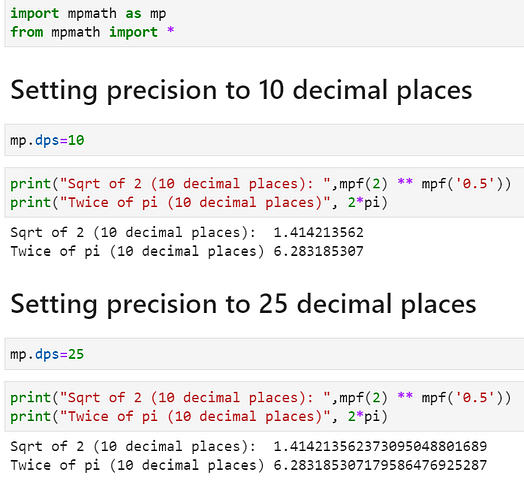You can print the `mp` context anytime to see the current global precision settings. The `mp.prec` shows the precision in bits and `mp.dps` shows in decimal.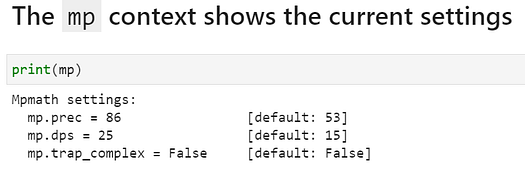## Use ``mpf`` instances instead of regular float

In the code snippets above you might have noticed a function `mpf`. An `mpf` instance holds a real-valued floating-point number. They work analogously to Python floats, but support arbitrary-precision arithmetic.

You should define `mpf` using strings (and not Python floats) as arguments to get true accuracy. You can also set `mp.pretty` to `True` for rounding w/o losing the internal accuracy.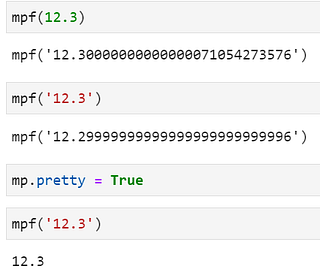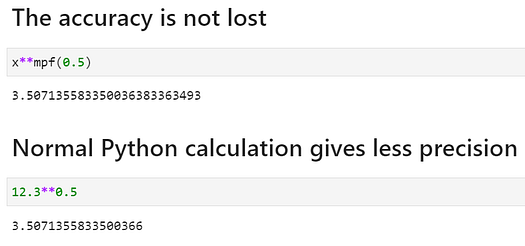## Now some magic! Factorial calculation 11,000 times faster

The `mpmath` can do large calculations using smart tricks whenever applicable. One example is factorial. For large numbers, it can use approximations appropriately without being instructed and give us the result much faster than the default Python math module.

Here is what happens when we attempt to calculate the factorial of 100,000. `mpmath` is 11,333X faster.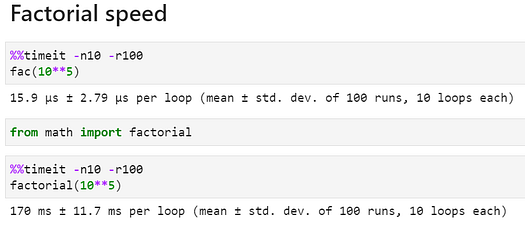## Rational and complex numbers are native citizens

We can throw in rational or complex numbers as easily as floating-point numbers into the mix. For this, we need to use a magic function `mpmathify` which works with `sympy` internals to interpret those quantities.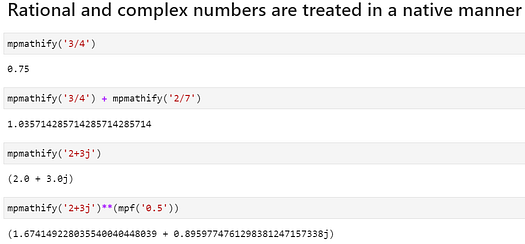We don’t have to import Python modules like `fraction` or `cmath` to work with such quantities at a precision of our choice.

Learn about all other general utility functions that `mpmath` offers here.

## Quick plotting

If Matplotlib is available on the system, `mpmath` offers a fast and easy plotting choice just by passing a list of functions and the corresponding ranges.

Here is a single-line code example,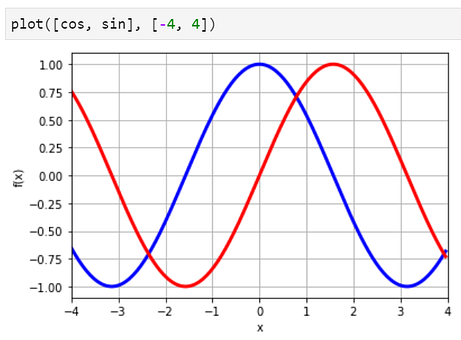Image source: Generated by the author

Another example with Fresnel functions,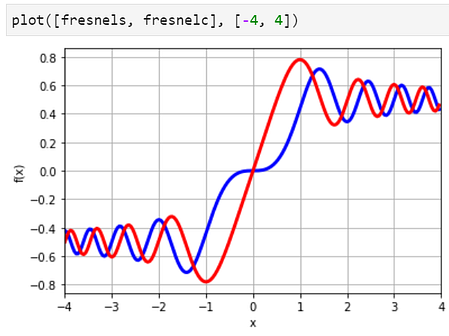Image source: Generated by the author

Complex quantities can be plotted as easily with `cplot`. By default, the complex argument (phase) is shown as color (hue) and the magnitude is shown as brightness.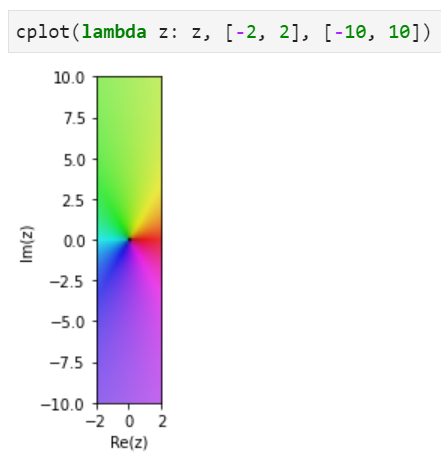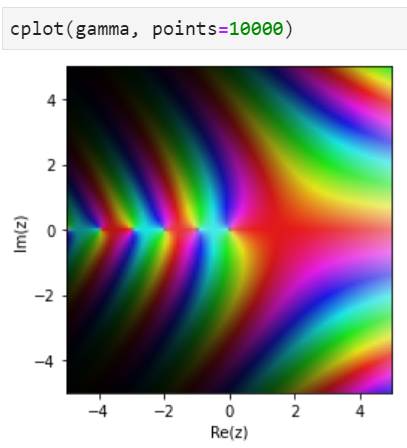Image source: Generated by the author

Surface plots are also game,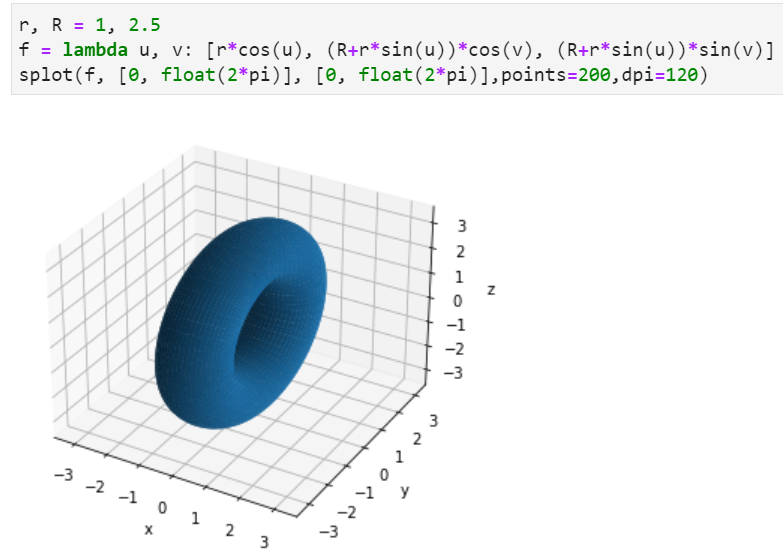Image source: Generated by the author

## Special functions

`mpmath` supports hundreds of special functions out-of-the-box. Here is a partial screenshot of that list. Refer to this documentation for details.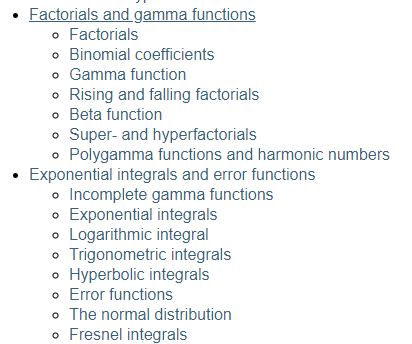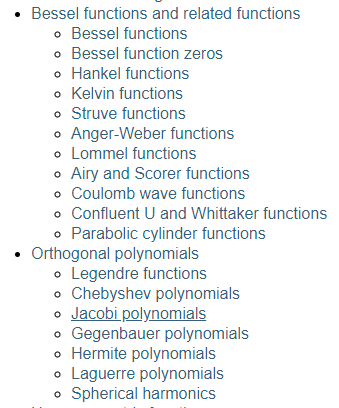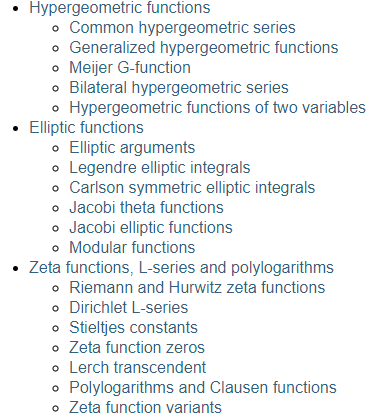Image source: Generated by the author

## Binomial coefficients

Fast and easy computation of binomial coefficients for statistics and combinatorial math with `binomial` function,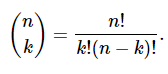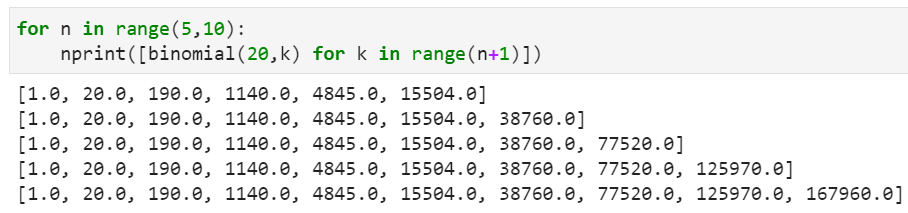This function supports massively large arguments, which is where this really shines. Scipy calculation is faster but for large numbers, Scipy does not even run!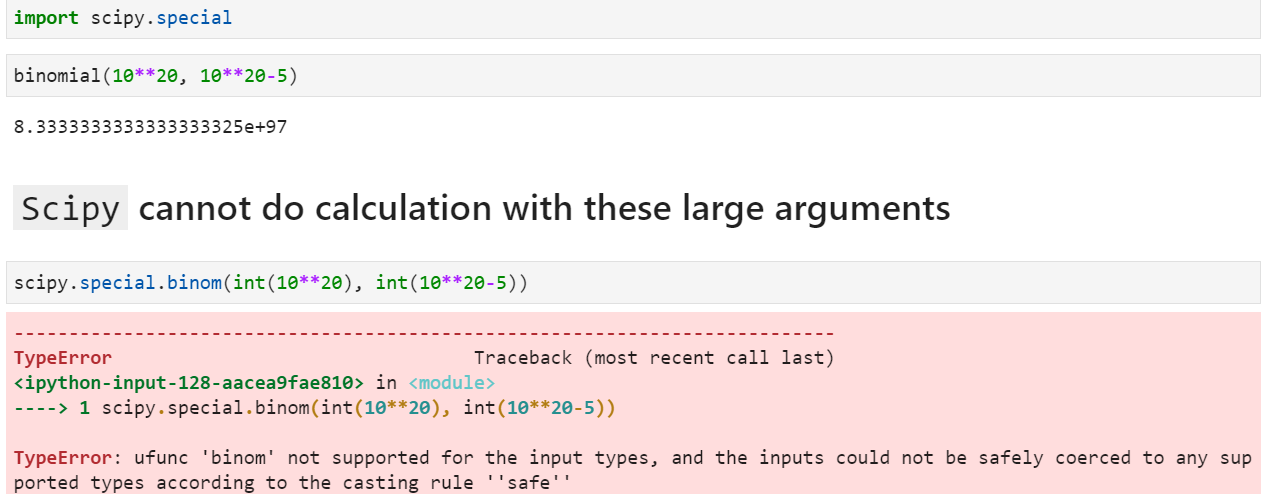And, extensions to non-integers and negative arguments are natural (using Gamma function),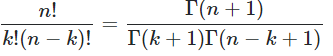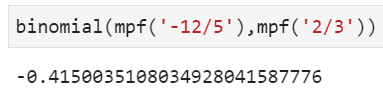## Hyperfactorials

For integers, hyperfactorials are defined as,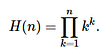They are really large. Can you think of an easy way to calculate this kind of number?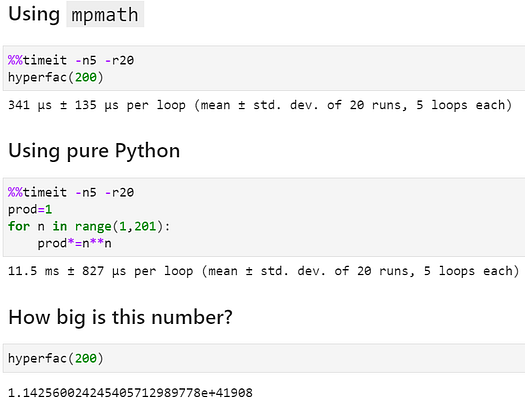This number is 1.1425…something followed by 41908 zeroes! To get an idea about that, just consider this fact — there are roughly 10⁸⁰ atoms in the universe i.e. 1 followed by 80 zeros.

Limitless math (in a fast manner), isn’t it?

## Fibonacci number

Calculating the Fibonacci sequence with naive Python (recursive function) is a popular interview question because it can be done in a few different ways which differ dramatically in efficiencies.

However, calculating approximate solutions with large arguments or non-integer (even complex) arguments is natural and easy for `mpmath`. This is not so straightforward to achieve using native Python coding.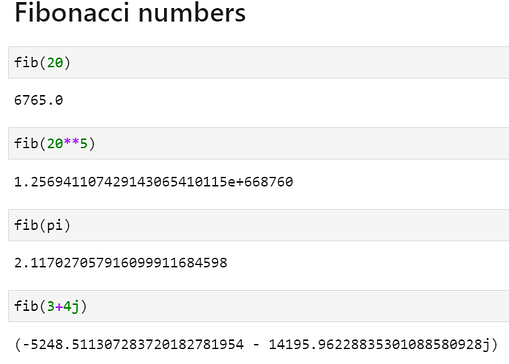Polynomial evaluation and roots

Easy and fast evaluation of polynomials of any order and root finding using `polyeval` and `polyroots` functions. Of course, `polyroots` evaluates all real and complex roots at one go.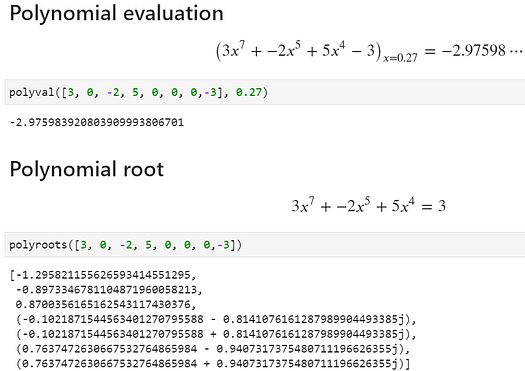## Root-finding of arbitrary functions

We can use the `rootfind` function to search for roots of any arbitrary function. Here is an example,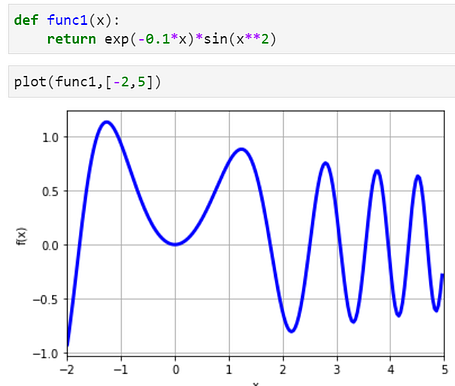Image source: Generated by the author

Then, we look for solutions near all the integers from -2 to 5 and it finds multiple solutions corresponding to all the values of x at which the function crosses zero.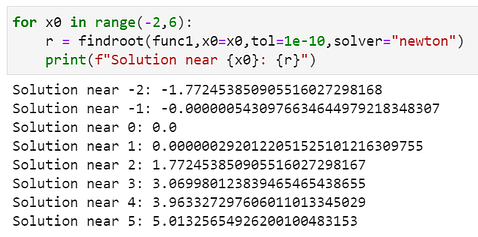## Numerical calculus

Evaluate derivatives of any order and any function,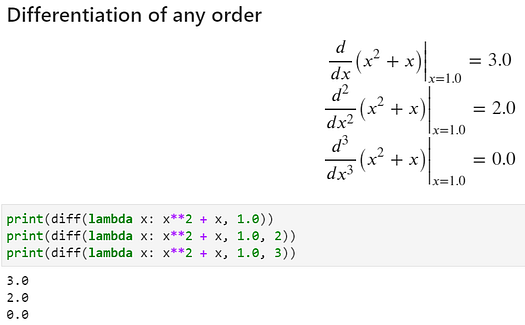Partial derivatives are easy,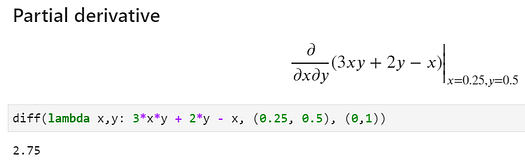Look at this reference to see other advanced examples and functions related to derivatives.

## 1-D integrals

Simple and fast evaluation to arbitrary precision,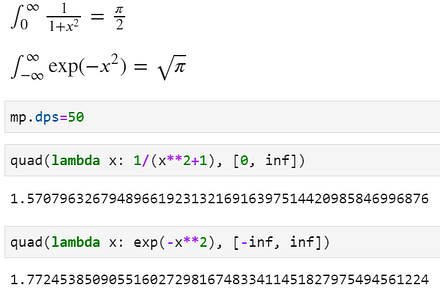## 2-D or 3-D integrals

Two- or three-dimensional integrals are also game!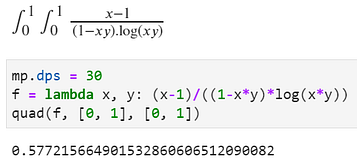Read more details here.

## Ordinary differential equation

We can use `odefun` to solve for ordinary differential equations with known boundary conditions.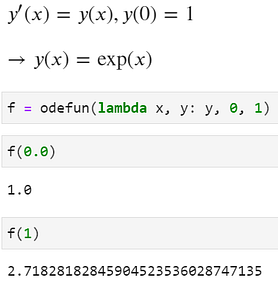Or, a more difficult one,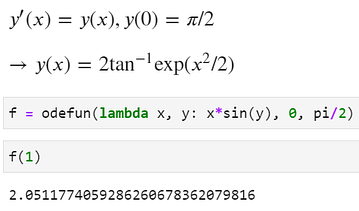## Matrix and linear algebra

The `mpmath` package also offers all the standard operations involving matrices and linear algebra. For brevity, we just refer to the documentation here instead of showing examples.

## Summary

In this article, we showed a powerful Python library and its capabilities for performing arbitrary-precision numerical computation involving all kinds of numbers and functions. Basically, the main advantage of this library is that it covers a very large swath of mathematical domains (algebraic, number-theory, calculus, special functions, etc.) and everything is available under a single roof without loading multiple libraries.

Many of the usages of this library are in complex scientific/ technological domains such as finite-element simulations or cryptography, but as a number-enthusiast, you can always pick up useful functions from this package whenever you need for your data science or machine learning work.

Again, here is the Notebook with all the code shown in this article.

Happy number crunching!

You can check the author’s GitHub repositories for code, ideas, and resources in machine learning and data science. If you are, like me, passionate about AI/machine learning/data science, please feel free to add me on LinkedIn or follow me on Twitter.

Bio: Tirthajyoti Sarkar is a Data Science/ML Manager at Adapdix Corp. He contributes regularly to publications such as KDnuggets and TDS on diverse topics related to data science and machine learning. He has authored data science books and contributes to open source software. Tirthajyoti holds a Ph.D. in EE and is working on an M.S. degree in Computational Data Analytics. Email him at tirthajyoti at gmail[dot]com.

Related:

By subscribing you accept KDnuggets Privacy Policy

By subscribing you accept KDnuggets Privacy Policy

By subscribing you accept KDnuggets Privacy Policy